/

### Tail Fitting of a Normal Distribution

One approach for fitting the tail of a distribution is to select an appropriate distributional family and then to select the parameters characterising the distribution in a manner that provides the best fit of the observed (cumulative) distribution function and/or quantile-quantile plot in the relevant tail.

Suppose that the observations are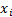for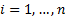. When ordered these are say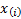. Weights given to each observation in the curve fitting process are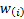. Typically we might expect theto be non-zero (and then typically constant) only forsufficiently small, or forsufficiently large, although this is not strictly necessary.

A common way of carrying out curve fitting is least squares, so a natural way of implementing this approach to fit a (univariate) Normal distribution to the data might be:

Any Normal distribution is characterised by a mean,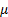, and standard deviation,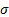. We might therefore derive,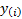, the expected value for the observation,  using the following formula: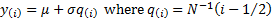[Note, the expected value of’th quantile of a Normal distribution is not precisely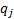as defined above because the pdf is not flat, see e.g. Expected Worst Loss Analysis]

We would then identify estimates of the mean,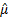, and standard deviation,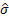, that together minimise the following least squares computation: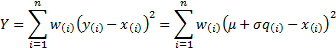This is minimised when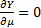and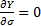, i.e. for the values ofandwhere: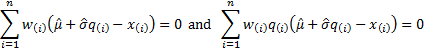If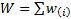,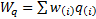,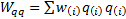,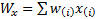and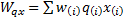then these equations simplify to: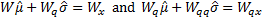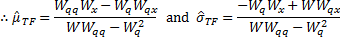Whilst this type of approach is primarily designed to be used merely in the tail of the distribution (i.e. withnon-zero, perhaps constant, only forsuitably small or, for the other tail, only forsuitably close to), we can also consider what answer this approach would give if it were applied to the entire distributional form, e.g. using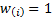for all. As the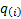are symmetric around 0.5, we have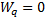so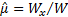, i.e.is then the usual maximum likelihood estimator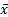. By, say, carrying out a simulation exercise we can also confirm thatis also typically close to the relevant maximum likelihood estimator ifis not very small.